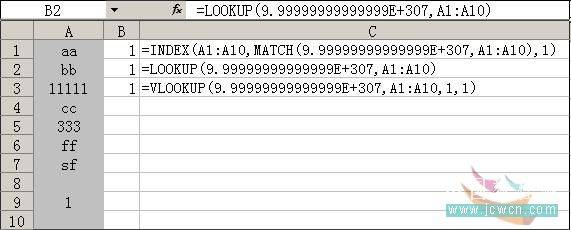# EXCEL 找出一组数据中的最后一个数值

Find the last numeric value in a range in Microsoft Excel
Text/Numeric data in column A
Formula in cell B1: =INDEX(A1:A10,MATCH(9.99999999999999E+307,A1:A10))
Result:1        (B1=1)

2.MATCH(lookup_value,lookup_array,match_type),如果省略 match_type，则假设为 1。

3. 除了用INDEX和MATCH函数，还可以用VLOOKUP或LOOKUP函数求出结果